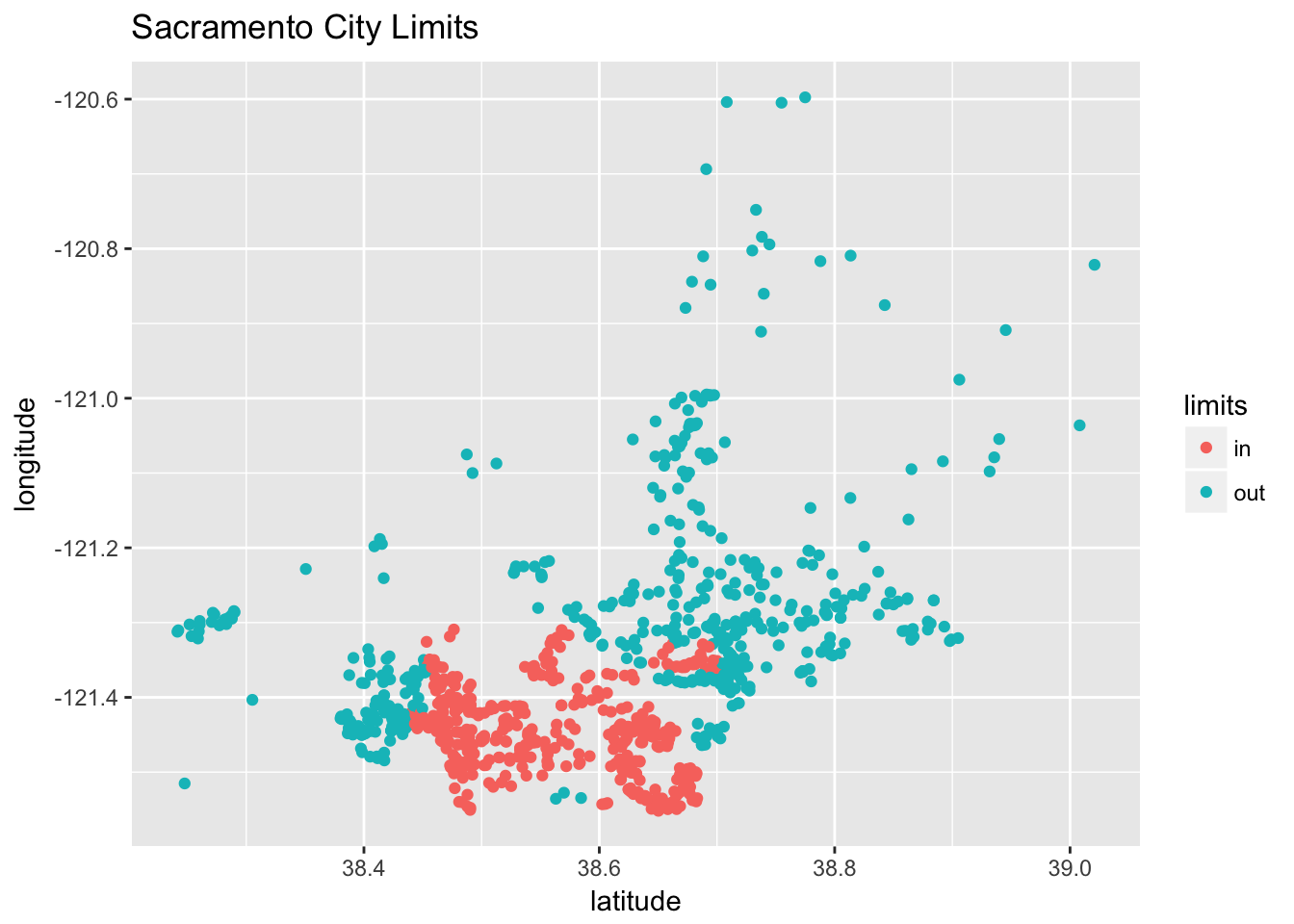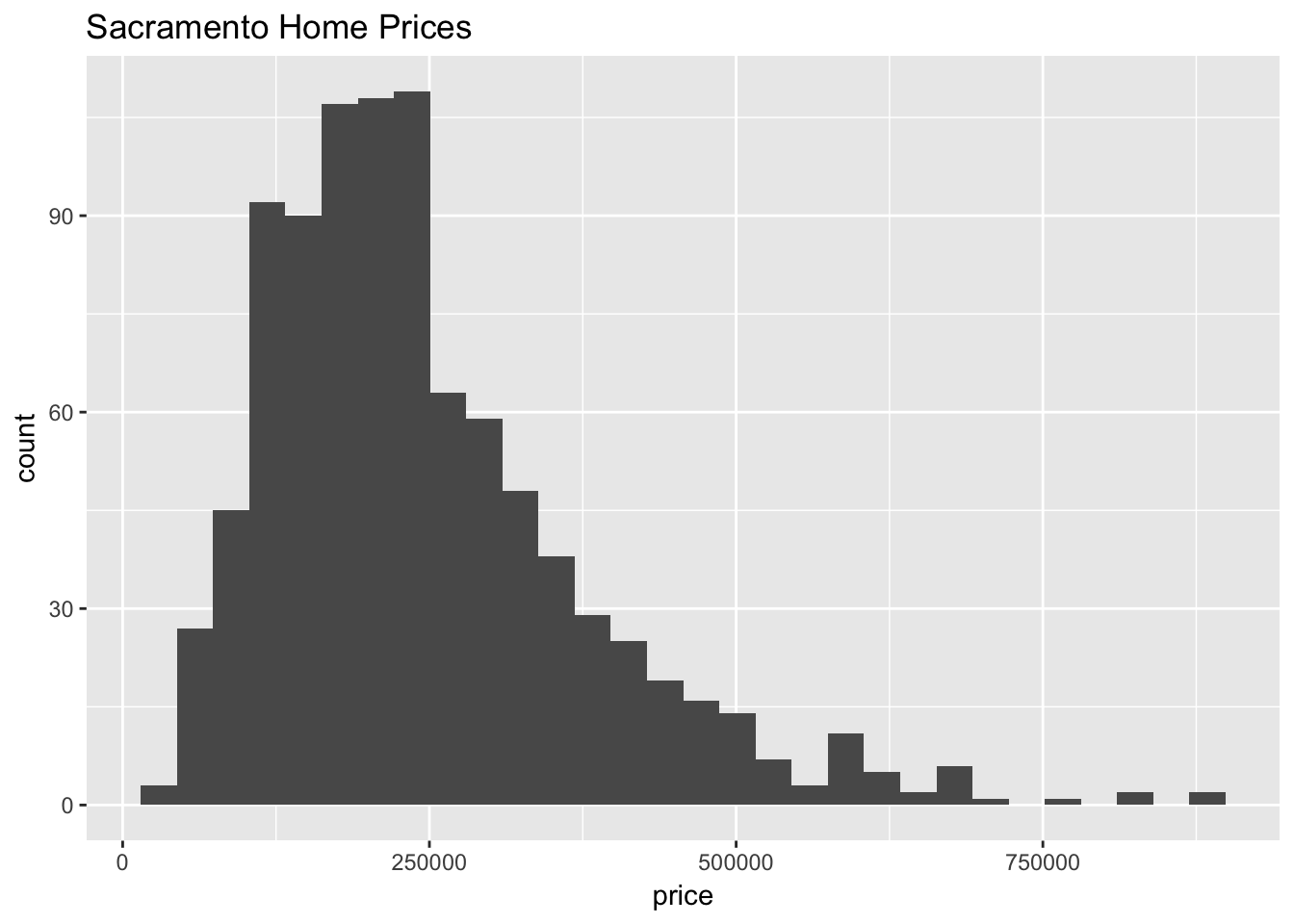For this homework we will use the Sacramento data from the caret package. You should read the documentation for this data. The goal of our modeling will be to predict home prices.

You may only use the following packages:

library(caret)
library(randomForest)
library(tidyverse)
library(knitr)
library(kableExtra)

Before modeling, we will perform some data preparation.

Instead of using the city or zip variables that exist in the dataset, we will simply create a variable indicating whether or not a house is technically within the city limits Sacramento. (We do this because they would both be factor variables with a large number of factors. This is a choice that is made due to laziness, not because it is justified. Think about what issues these variables might cause.)

data(Sacramento)
sac_data = Sacramento
sac_data$limits = factor(ifelse(sac_data$city == "SACRAMENTO", "in", "out"))
sac_data = subset(sac_data, select = -c(city, zip))

A plot of longitude versus latitude gives us a sense of where the city limits are.

qplot(y = longitude, x = latitude, data = sac_data,
col = limits, main = "Sacramento City Limits ")You should consider performing some additional exploratory data analysis, but we provide a histogram of the home prices.

qplot(x = price, data = sac_data, main = "Sacramento Home Prices")After these modifications, we test-train split the data.

set.seed(42)
sac_trn_idx  = sample(nrow(sac_data), size = trunc(0.80 * nrow(sac_data)))
sac_trn_data = sac_data[sac_trn_idx, ]
sac_tst_data = sac_data[-sac_trn_idx, ]

The training data should be used for all model fitting.

## Exercise 1 (Modeling Price, Without Location)

For this exercise, we will create linear models in an attempt to be able to predict price, without the use of the limits, latitude, or longitude variables. Do not modify sac_trn_data.

With the available variables, fit the following models:

• An additive model using all availible predictors
• A model using only sqft as a predictor
• $$\texttt{price} = \beta_0 + \beta_1 \texttt{sqft} + \beta_2 \texttt{multi} + \beta_3 \texttt{res} + \epsilon$$
• $$\texttt{price} = \beta_0 + \beta_1 \texttt{sqft} + \beta_2 \texttt{multi} + \beta_3 \texttt{res} + \beta_4 (\texttt{sqft}\times\texttt{multi}) + \beta_5 (\texttt{sqft}\times\texttt{res}) + \epsilon$$

Here, res is a dummy variable indicating whether or not type is Residential. Similarly, multi is a dummy variables indicating whether or not type is Multi_Family.

Summarize these models using a well-formatted markdown table which includes columns for:

• Model (written using R’s formula syntax)
• Train RMSE
• Test RMSE

Solution:

# fit models
sac_mod_1 = lm(price ~ . -limits -latitude -longitude, data = sac_trn_data)
sac_mod_2 = lm(price ~ sqft, data = sac_trn_data)
sac_mod_3 = lm(price ~ sqft + type, data = sac_trn_data)
sac_mod_4 = lm(price ~ sqft * type, data = sac_trn_data)
# helper function for RMSE
calc_rmse = function(actual, predicted) {
sqrt(mean((actual - predicted) ^ 2))
}
# create model list
e1_mod_list = list(sac_mod_1, sac_mod_2, sac_mod_3, sac_mod_4)

# get predictions
e1_trn_pred = lapply(e1_mod_list, predict, newdata = sac_trn_data)
e1_tst_pred = lapply(e1_mod_list, predict, newdata = sac_tst_data)

# get RMSEs
e1_trn_rmse = sapply(e1_trn_pred, calc_rmse, actual = sac_trn_data$price) e1_tst_rmse = sapply(e1_tst_pred, calc_rmse, actual = sac_tst_data$price)
Model Train RMSE Test RMSE
price ~ . -limits -latitude -longitude 82661 78852
price ~ sqft 84713 81337
price ~ sqft + type 84169 81214
price ~ sqft * type 83517 81096

## Exercise 2 (Modeling Price, With Location)

For this exercise, we will create models in an attempt to be able to predict price, using all available predictors.

Fit a total of four models:

• add: an additive linear model using all availible predictors
• int: a linear model using all the main effects of all availible predictors as well, as all possible two-way interactions
• user: a linear model that performs better than add and int
• rf: a random forest which uses all available predictors fit using the randomForest() function from the randomForest package with all default arguments. To specify the predictors used, use the formula syntax for an additive model

Summarize these models using a well-formatted markdown table which includes columns for:

• Model Name
• Model Type (lm or rf)
• Variables Used (may use formula syntax)
• Train RMSE
• Test RMSE

Solution:

# fit models
sac_mod_add  = lm(price ~ ., data = sac_trn_data)
sac_mod_int  = lm(price ~ . ^ 2, data = sac_trn_data)
sac_mod_user = lm(price ~ . + sqft:type + type:limits - baths, data = sac_trn_data)
sac_mod_rf   = randomForest(price ~ ., data = sac_trn_data)
# create model list
e2_mod_list = list(sac_mod_add, sac_mod_int, sac_mod_user, sac_mod_rf)

# get predictions
e2_trn_pred = lapply(e2_mod_list, predict, newdata = sac_trn_data)
e2_tst_pred = lapply(e2_mod_list, predict, newdata = sac_tst_data)

# get RMSEs
e2_trn_rmse = sapply(e2_trn_pred, calc_rmse, actual = sac_trn_data$price) e2_tst_rmse = sapply(e2_tst_pred, calc_rmse, actual = sac_tst_data$price)
Model Name Model Type Variables Used Train RMSE Test RMSE
add lm . 79575 73255
int lm . ^ 2 75771 74598
user lm . + sqft:type + type:limits - baths 79304 72860
rf rf . 44743 69313

Note: The user model was found via trial-and-error. The first interaction was somewhat suggested via the results of Exercise 1. The removal of baths is due to its high correlation with beds. More on why that correlation reduces prediction later…

## Exercise 3 (Modeling Price, Response Transformation)

Re-fit each of the models from Exercise 2, but with a log transformation applied to the response. (Do not modify the data to do so.)

Summarize the results of these four models using a well-formatted markdown table which includes columns for:

• Mode Name (append log_ to the start of the previous names)
• Model Type (lm or rf)
• Variables Used (may use formula syntax)
• Train RMSE (on the data scale, that is, units of dollars)
• Test RMSE (on the data scale, that is, units of dollars)

Solution:

# fit models
sac_mod_log_add  = lm(log(price) ~ ., data = sac_trn_data)
sac_mod_log_int  = lm(log(price) ~ . ^ 2, data = sac_trn_data)
sac_mod_log_user = lm(log(price) ~ . + sqft:type + type:limits - baths, data = sac_trn_data)
sac_mod_log_rf   = randomForest(log(price) ~ ., data = sac_trn_data)
# create model list
e3_mod_list = list(sac_mod_log_add, sac_mod_log_int, sac_mod_log_user, sac_mod_log_rf)

# get predictions
e3_trn_pred = lapply(e3_mod_list, predict, newdata = sac_trn_data)
e3_tst_pred = lapply(e3_mod_list, predict, newdata = sac_tst_data)

# transform predictions to original scale
e3_trn_pred = lapply(e3_trn_pred, exp)
e3_tst_pred = lapply(e3_tst_pred, exp)

# get RMSEs
e3_trn_rmse = sapply(e3_trn_pred, calc_rmse, actual = sac_trn_data$price) e3_tst_rmse = sapply(e3_tst_pred, calc_rmse, actual = sac_tst_data$price)
Model Name Model Type Variables Used Train RMSE Test RMSE
log_add lm . 88166 86134
log_int lm . ^ 2 79105 76994
log_user lm . + sqft:type + type:limits - baths 87520 84396
log_rf rf . 50005 71468

## Exercise 4 (Concept Checks)

[a] Which model in Exercise 1 performs best?

Solution: The additive model, as it obtains the lowest test RMSE.

[b] Which model in Exercise 2 performs best?

Solution: The random forest model, as it obtains the lowest test RMSE.

[c] Which model in Exercise 3 performs best?

Solution: The random forest model, as it obtains the lowest test RMSE.

[d] Does the log transformation appear justified? Explain.

Solution: NO! The models which use the log transformation all perform worse than without the transformation. The skewed histogram is not sufficient justification for the transformation, only an indication that it should be tried.

[e] Does location appear to be helpful for predicting price? Explain.

Solution: Yes. The models which use locations information appear to provided better predictive performance. (Although, to be sure, we should have tried a random forest without the location information.)

[f] Suggest a reason for performing this analysis. The reason we are creating the models is to predict price. From an analysis perspective, why might these predictions be useful?

Solution: We could use our model to set an asking price when selling a house, without the need for a realtor.

[g] With your answer to part [f] in mind, is the best model we found at all useful? Explain.

Solution: Probably not! Our best model achieves a test RMSE of 69313. That is about a third of the median home price, 220000! We should probably get a realtor! Perhaps the analysis should be done on “regular” houses, and “luxury” homes separately. (Or, should we evaluate RMSE for only “regular” houses to see if our model is actually predicting well in that range? Maybe the errors for the “luxury” homes is driving up our RMSE…)